# ML Aggarwal Class 10 Solutions for ICSE Maths Chapter 9 Arithmetic and Geometric Progressions Ex 9.3

## ML Aggarwal Class 10 Solutions for ICSE Maths Chapter 9 Arithmetic and Geometric Progressions Ex 9.3

ML Aggarwal Class 10 Solutions for ICSE Maths Chapter 9 Arithmetic and Geometric Progressions Ex 9.3

Question 1.
Find the sum of the following A.P.s:
(i) 2, 7, 12, … to 10 terms
(ii) $$\frac { 1 }{ 15 } ,\frac { 1 }{ 12 } ,\frac { 1 }{ 10 } ,…$$ t0 11 terms
Solution: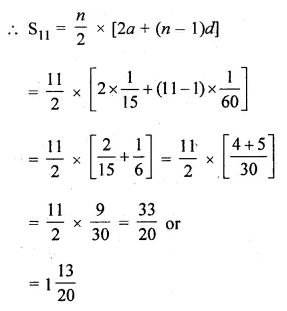Question 2.
How many terms of the A.P. 27, 24, 21, …, should be taken so that their sum is zero?
Solution:Question 3.
Find the sums given below :
(i) 34 + 32 + 30 + … + 10
(ii) -5 + ( -8) + ( -11) + … + ( -230)
Solution: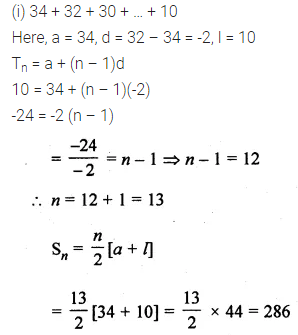Question 4.
In an A.P. (with usual notations) :
(i) given a = 5, d = 3, an = 50, find n and Sn
(ii) given a = 7, a13 = 35, find d and S13
(iii) given d = 5, S9 = 75, find a and a9
(iv) given a = 8, an = 62, Sn = 210, find n and d
(v) given a = 3, n = 8, S = 192, find d.
Solution: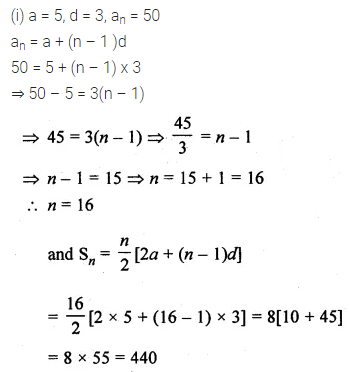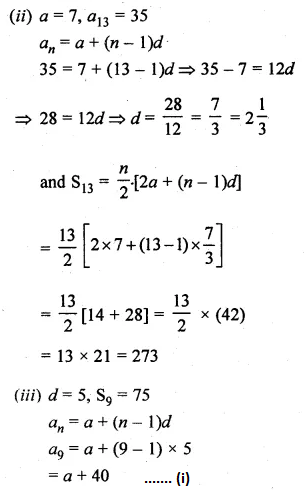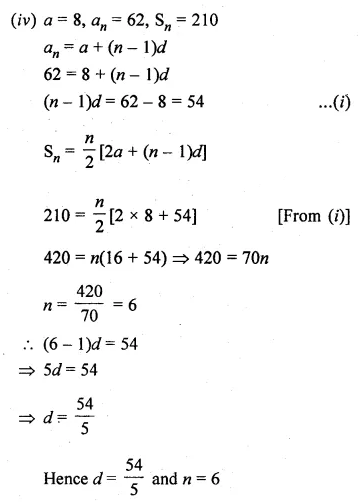Question 5.
(i) The first term of an A.P. is 5, the last term is 45 and the sum is 400. Find the number of terms and the common difference.
(ii) The sum of the first 15 terms of an A.P. is 750 and its first term is 15. Find its 20th term.
Solution: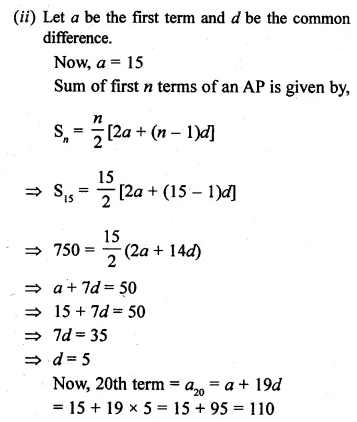Question 6.
The first and the last terms of an A.P. are 17 and 350 respectively. If the common difference is 9, how many terms are there and what is their sum?
Solution: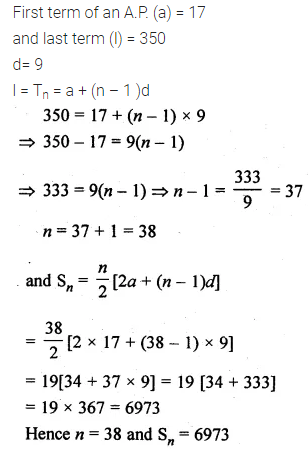Question 7.
Solve for x : 1 + 4 + 7 + 10 + … + x = 287.
Solution: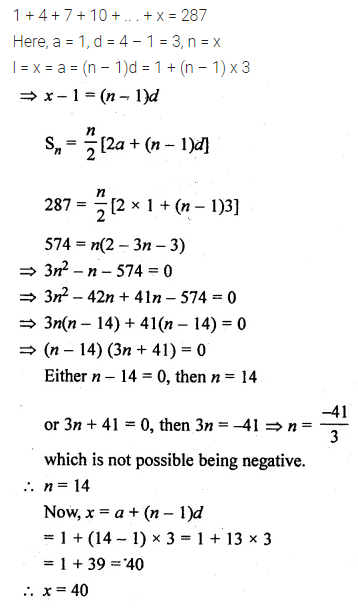Question 8.
(i) How many terms of the A.P. 25, 22, 19, … are needed to give the sum 116? Also, find the last term.
(ii) How many terms of the A.P. 24, 21, 18, … must be taken so that the sum is 78? Explain the double answer.
Solution: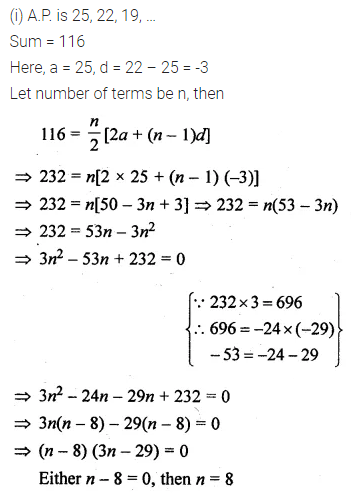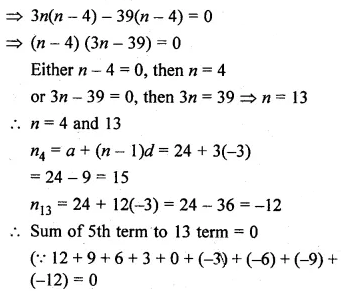Question 9.
Find the sum of first 22 terms, of an A.P. in which d = 7 and a22 is 149.
Solution:Question 10.
(i) Find the sum of the first 51 terms of the A.P. whose second and third terms are 14 and 18 respectively.
(ii) If the third term of an A.P. is 1 and 6th term is -11, find the sum of its first 32 terms.
Solution: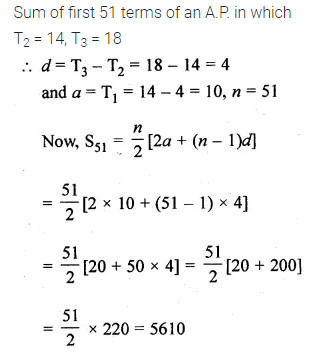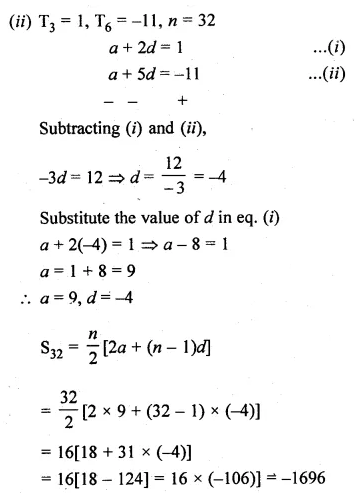Question 11.
If the sum of the first 6 terms of an A.P. is 36 and that of the first 16 terms is 256, find the sum of the first 10 terms.
Solution: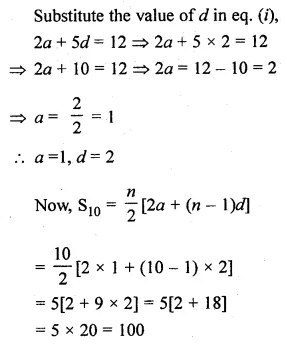Question 12.
Show that a1, a2, a3, … form an A.P. where an is defined as an = 3 + 4n. Also, find the sum of the first 15 terms.
Solution: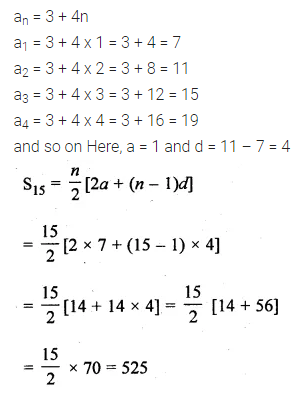Question 13.
(i) If an = 3 – 4n, show that a1, a2, a3, … form an A.P. Also find S20.
(ii) Find the common difference of an A.P. whose first term is 5 and the sum of the first four terms is half the sum of the next four terms.
Solution:Question 14.
The sum of first n terms of an A.P. whose first term is 8 and the common difference is 20 equal to the sum of first 2n terms of another A.P. whose first term is -30 and the common difference is 8. Find n.
Solution: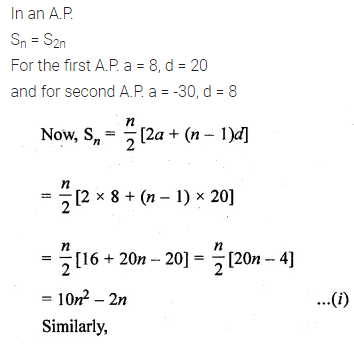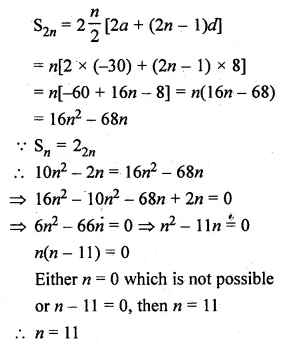Question 15.
The sum of the first six terms of an arithmetic progression is 42. The ratio of the 10th term to the 30th term is $$\\ \frac { 1 }{ 3 }$$. Calculate the first and the thirteenth term.
Solution:Question 16.
In an A.P., the sum of its first n terms is 6n – n². Find the 25th term.
Solution:Question 17.
If the sum of first n terms of an A.P. is 4n – n², what is the first term (i. e. S1)? What is the sum of the first two terms? What is the second term? Also, find the 3rd term, the 10th term, and the nth terms?
Solution:Question 18.
If Sn denotes the sum of first n terms of an A.P., prove that S30 = 3(S20 – S10).
Solution: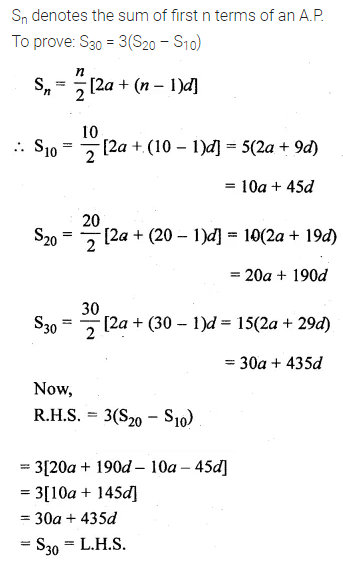Question 19.
(i) Find the sum of the first 1000 positive integers.
(ii) Find the sum of first 15 multiples of 8.
Solution:Question 20.
(i) Find the sum of all two digit natural numbers which are divisible by 4.
(ii) Find the sum of all natural numbers between 100 and 200 which are divisible by 4.
(iii) Find the sum of all multiples of 9 lying between 300 and 700.
(iv) Find the sum of all natural numbers less than 100 which are divisible by 6.
Solution: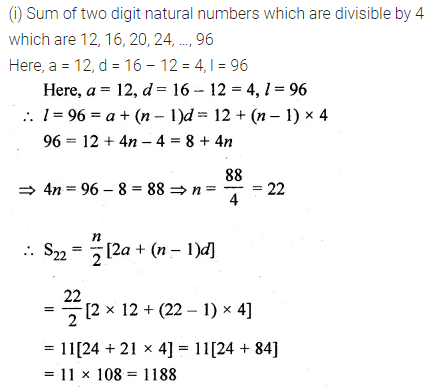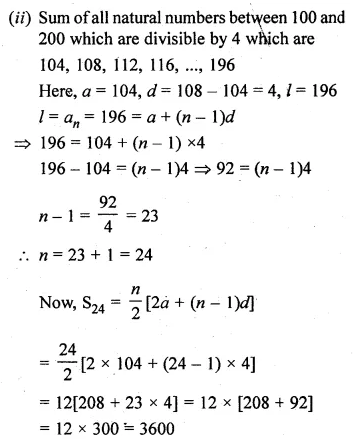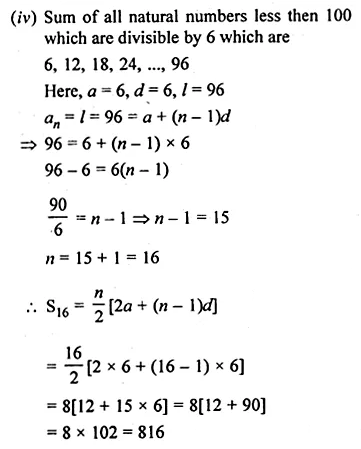Question 21.
(i) Find the sum of all two digit odd positive numbers.
(ii) Find the sum of all 3-digit natural numbers which are divisible by 7.
(iii) Find the sum of all two digit numbers which when divided by 7 yields 1 as the remainder.
Solution: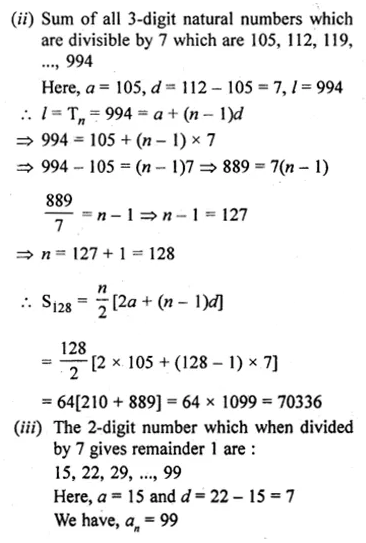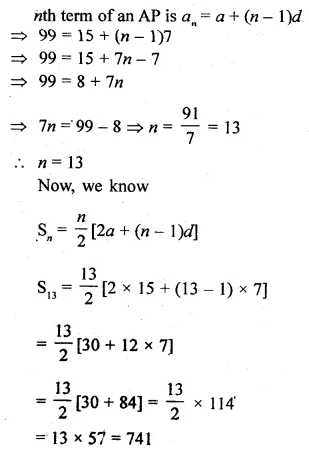Question 22.
A contract on construction job specifies a penalty for delay of completion beyond a certain date as follows: Rs 200 for the first day, Rs 250 for the second day, Rs 300 for the third day, etc., the penalty for each succeeding day being Rs 50 more than for the preceding day. How much money the contractor has to pay a penalty if he has delayed the work for 30 days?
Solution: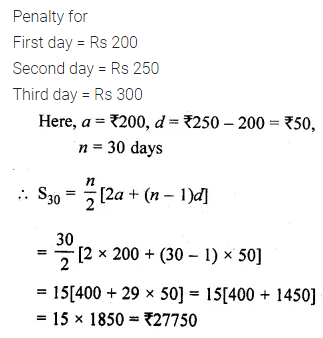Question 23.
Kanika was given her pocket money on 1st Jan 2016. She puts Rs 1 on Day 1, Rs 2 on Day 2, Rs 3 on Day 3, and continued on doing so till the end of the month, from this money into her piggy bank. She also spent Rs 204 of her pocket money and was found that at the end of the month she still has Rs 100 with her. How much money was her pocket money for the month?
Solution: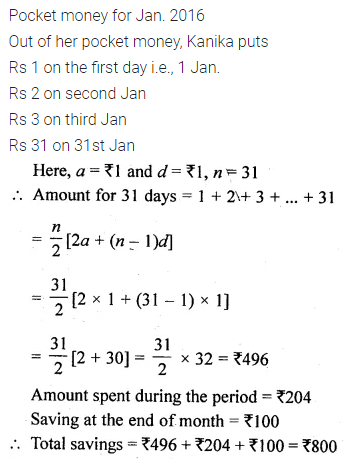Question 24.
Yasmeen saves Rs 32 during the first month, Rs 36 in the second month and Rs 40 in the third month. If she continues to save in this manner, in how many months will she save Rs 2000?
Solution:Question 25.
The students of a school decided to beautify the school on the Annual Day by fixing colourful flags on the straight passage of the school. They have 27 flags to be fixed at intervals of every 2 m. The flags are stored at the position of the middle most flag. Ruchi was given the responsibility of placing the flags. Ruchi kept her books where the flags were stored. She could carry only one flag at a time. How much distance did she cover in completing this job and returning back to collect her books? What is the maximum distance she traveled carrying a flag?
Solution: